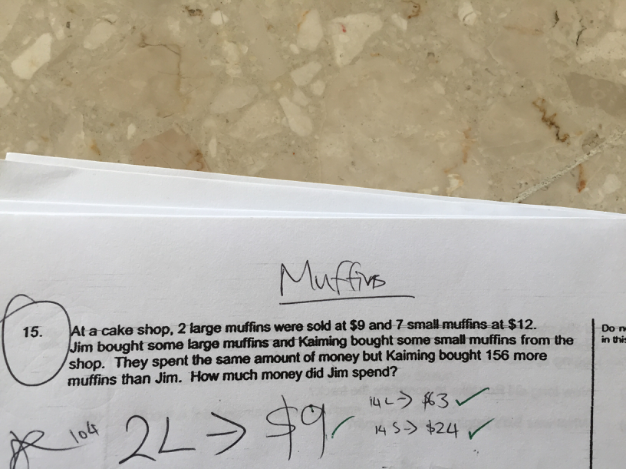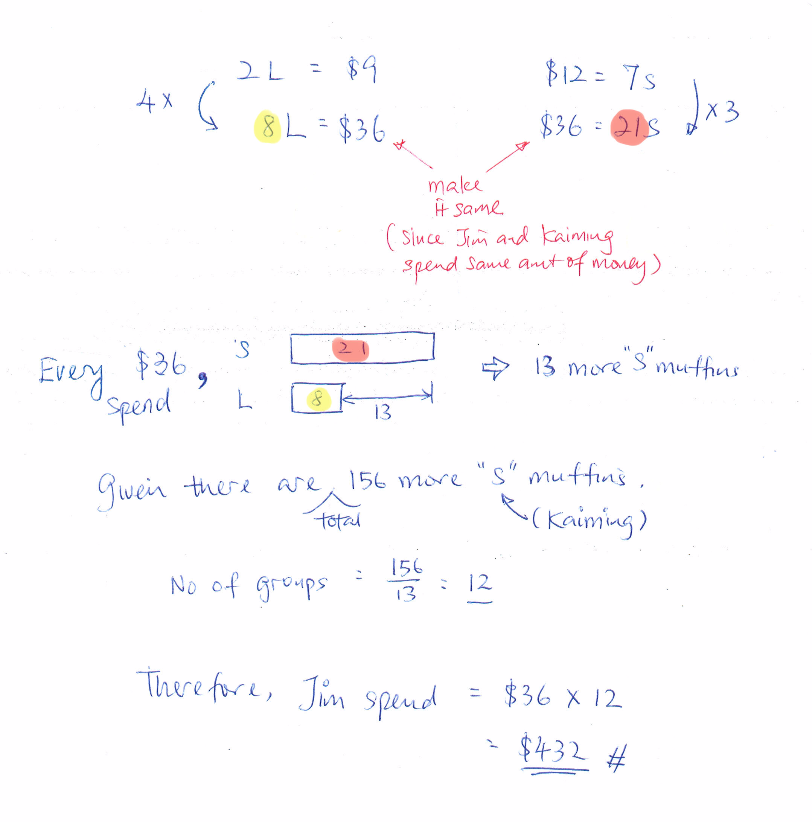QuestionHi ,

pls help of the above question .

Thanks  very much in advance !

regards

Fly2High

Source: Pei Chun Public

Let ‘u’ be the number of sets of large muffin bought and ‘p’ be the number of set of small muffins bought

They spent the same amount
9u = 12p => 18u = 24p

Small muffin is 156 more than large muffins
7p = 2u + 156 => 63p = 18u + 1404

Substitute 18u =24p into the above equation

63p = 24p + 1404
39p = 1404
p = 36

Kaiming spent 12p = \$432 which is also the same amount Jim spent.

0 Replies 2 Likes ✔Accepted AnswerJust another alternative.

0 Replies 1 Like